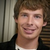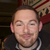+1

# Is there something different I need to do to handle negative numbers in if statements?

thillsman 8 years ago updated 8 years ago
I'm coming across a weird issue. I've got an eval statement calculating [var:firstnumber][var:operator][var:secondnumber] where firstnumber and secondnumber are both integers and operation is either + or -.

By using an alert, I can see what the numerical output of the eval statement is, and it's always correct. However, I have code like this:

``` <if lhs="[var:answer]" operator="e" rhs="4"> <assign property="object:ANSWER.text" value="four" /> </if> <if lhs="[var:answer]" operator="e" rhs="-4"> <assign property="object:ANSWER.text" value="negative four" /> </if> ```

What's weird is that the wire puts "four" as the text value in the first instance, but doesn't put "negative four" in the second. In either instance, the alert box showing [var:answer] will show the correct answer: 4 or -4 depending on the situation.Are you embedding the alert into the if statement to confirm that the "if lhs="[var:answer]" operator="e" rhs="-4" is rendering true?Good call! I put the alert within the if statement, just before the < assign> action. It returns -4 in the alert box, but doesn't write anything to object:ANSWER.text. So there's still an issue but it's not the < if> action's fault. Weird.Make sure your ANSWER text is long enough and tall enough to display the extra characters. It might be too small to display "negative four", but big enough to display "four"Genius! Of course. Good guess. The panel the text lives in was too small. Thanks, Ian!